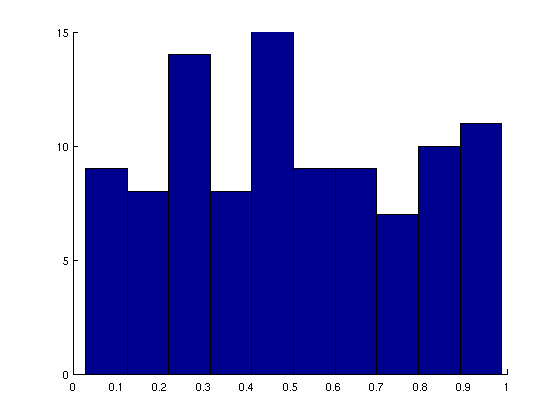# plotting without certain axes in matplotlib

hi all,

i’m trying to generate a very simple plot, where only the left y axis and the bottom x axis are present. i.e. there is no top x axis or right y axis… this is the default for many plotting packages. in matlab, one can do this as follows:

x = rand(1,100);
hist(x)
set(gca, ‘Box’, ‘off’, ‘LineWidth’, 1);

so the set(gca, ‘Box’, ‘off’…) command does the job. what’s the analogous matplotlib command? i would like to do this preferably without any external packages.

I attach the figure so you can see what i mean…

thank you.per freem wrote:

hi all,

i'm trying to generate a very simple plot, where only the left y axis and the bottom x axis are present. i.e. there is no top x axis or right y axis... this is the default for many plotting packages. in matlab, one can do this as follows:

>> x = rand(1,100);
>> hist(x)
>> set(gca, 'Box', 'off', 'LineWidth', 1);

so the set(gca, 'Box', 'off'...) command does the job. what's the analogous matplotlib command? i would like to do this preferably without any external packages.

There is no analogous command, despite many requests such as yours. Recipes for getting this effect have been posted to the list, though. See also http://matplotlib.sourceforge.net/examples/pylab_examples/manual_axis.html.

Making axes more flexible, to handle your case and others such as axes crossing in the middle of a figure, is on the wish list, but turns out to require quite a bit of work--more than one might expect.

Eric